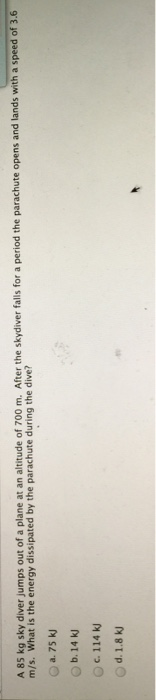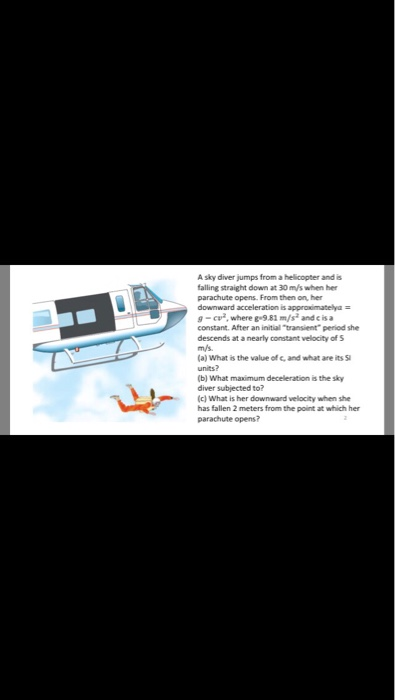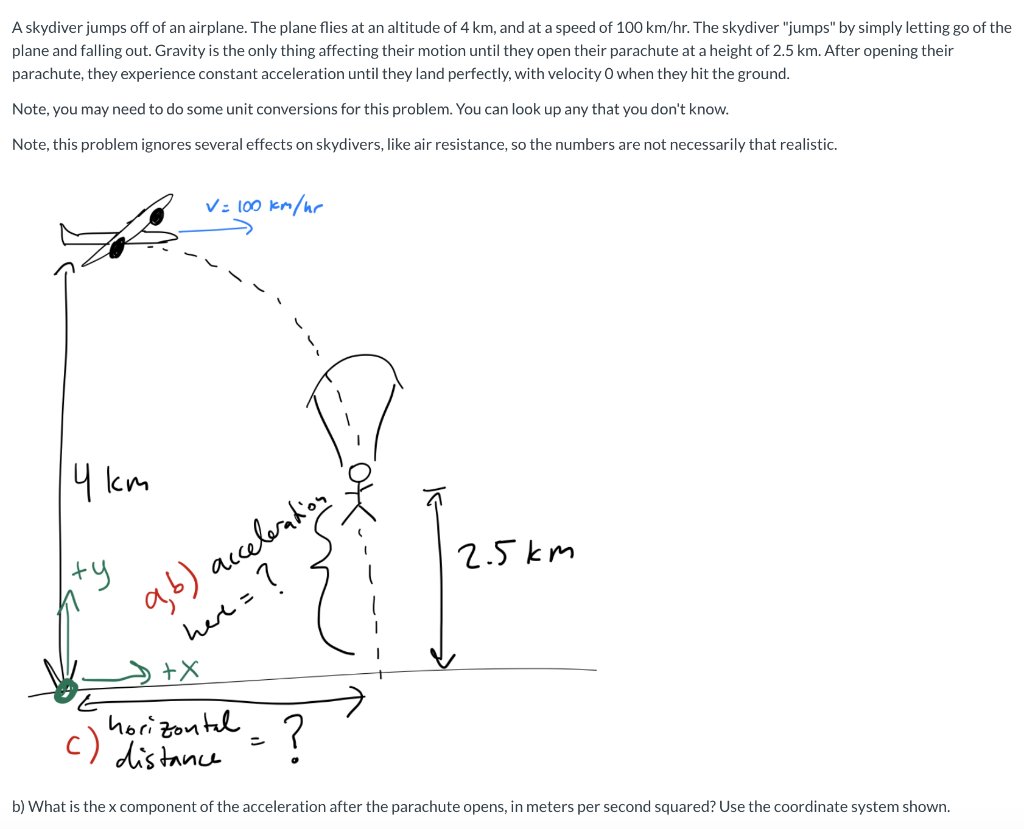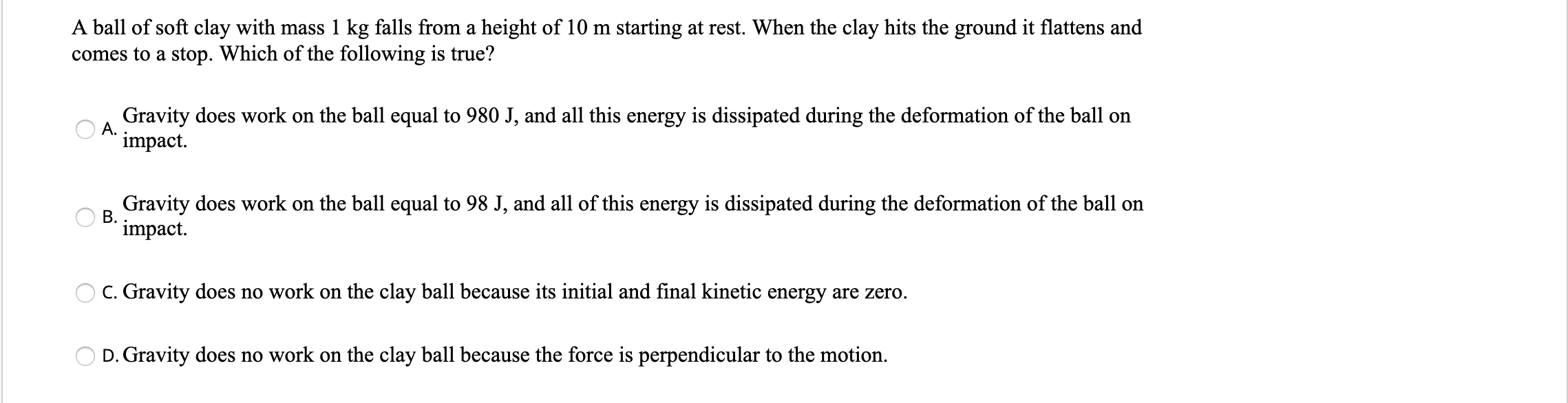Question

A 85 kg sky diver jumps out of a plane at an altitude of 700 m....A 85 kg sky diver jumps out of a plane at an altitude of 700 m. After the skydiver falls for a period the parachute opens and lands with a speed of 3.6 m/s. What is the energy dissipated by the parachute during the dive? 75 kJ 14 kJ 114 kJ 1.8 kJ

If the parachute had not been used, landing speed would have been,

v = (2gh)1/2 = (2 * 9.81 * 700)1/2 = 117.2 m/s

Energy dissipated, E = 85 * (117.22 - 3.62) / 2 = 583 kJ

SOLUTION :

P. E. lost on reaching the ground

= m g h

= 85 * 9.8 * 700

= 583100 J

K. E. of the driver at  the time of landing

= 1/2 m v^2

= 1/2 * 85 * (3.6)^2

= 550.8 J

Energy dissipated by the parachute during dive

= 583100 - 550.8

= 582549.2 J

Earn Coins

Coins can be redeemed for fabulous gifts.

Similar Homework Help Questions
• An 82.0 kg skydiver jumps out of a balloon at an altitude of 1 500 m and opens the parachute at an altitude of 195 m

An 82.0 kg skydiver jumps out of a balloon at an altitude of 1 500 m and opens the parachute at an altitude of 195 m. (a) Assuming that the total retarding force on the diver is constant at 55.0 N with the parachute closed and constant at 3 600 N with the parachute open, what is the speed of the diver when he lands on the ground?

• A sky diver jumps from a helicopter and is falling straight down at 30 m/s when...A sky diver jumps from a helicopter and is falling straight down at 30 m/s when her parachute opens. From then on, her downward acceleration is approximately = g - cv^2, where g = 9.81 m/s^2 and c is a constant. After an initial "transient" period the descends at a nearly constant velocity of 5 m/s. (a) What is the value of c, and what are its 9 units? (b) What maximum deceleration is the sky diver subjected to? (c)...

• Retarding force

12. An 80.0 kg skydiver jumps out of a balloon at an altitude of1060 m and opens the parachute at analtitude of 170 m. (a) Assuming thatthe total retarding force onthe diver is constant at 50.0 N withthe parachute closed and constant at 3600 N with the parachuteopen, what is the speed of the diver when he lands ontheground?1 m/s(b) Do you think the sky diver will be injured? Explain.2(c) At what height should the parachute be opened so that...

• A 3900 kg car is parked at the top of an 8.7 m long driveway that...

A 3900 kg car is parked at the top of an 8.7 m long driveway that is sloped at 17? with the horizontal. The parking brake fails. If an average friction force of 4200 N im- pedes the motion, find the speed of the car at the bottom of the driveway. The acceleration of gravity is 9.8 m/s2 . Starting from rest, a 10.0 kg suitcase slides 3.00 m down a frictionless ramp inclined at 31.0? from the floor. The...

• A sky diver jumps out of an airplane and after dropping a distance of 46 m,...

A sky diver jumps out of an airplane and after dropping a distance of 46 m, the diver has lost 25000.0 J of gravitational potential energy. What is the gravitational force acting on the diver? between 700 and 750 N between 600 and 650 N between 850 and 900 N between 550 and 600 N between 650 and 700 N less than 550 N greater than 900 N between 750 and 800 N

Dear Cramster Friends,Below is a challenge that I encountered but to which theanswer is not given. Can you please see if I have attemptedcorrectly? I urge you to please not be put offby the lenght of thequestion. Whilst I don't deny that the question has too many parts,the truth of the matter is that it won't take long at all.Thank-you verymuch for your time and effort.(Q) A sky diver of mass 80.0 (including parachute) jumps off a plane and begins...

• A parachutist jumps out of a plane at an altitude of 3000 meters. The parachutist unfolds...

A parachutist jumps out of a plane at an altitude of 3000 meters. The parachutist unfolds his parachute when he reaches a height of 800 m. Then the parachute is unfolded, the parachutist will: (a) continue to fall downwards but the speed quickly becomes significantly slower; (b) stop for a moment, and then continue to fall, but slower than before the parachute unfolded; (c) go up for a moment, and then continue to fall, but slower than before the parachute...

• Note that the answer is not: - 0 m/s^2 - 7.848 m/s^2 A skydiver jumps off...Note that the answer is not: - 0 m/s^2 - 7.848 m/s^2 A skydiver jumps off of an airplane. The plane flies at an altitude of 4 km, and at a speed of 100 km/hr. The skydiver "jumps" by simply letting go of the plane and falling out. Gravity is the only thing affecting their motion until they open their parachute at a height of 2.5 km. After opening their parachute, they experience constant acceleration until they land perfectly, with...

• A 85 kg trampoline artist jumps vertically upward from the top of a platform with a speed of 3.5 m/s

A 85 kg trampoline artist jumps vertically upward from the top of a platform with a speed of 3.5 m/s. How fast is he going as he lands on the trampoline, 3.0 m below? If the trampoline behaves like a spring of spring constant 5.2 x 10^4 N/m, how far does he depress it? My professor has not discussed any problem similar to this one, so I do not know where to begin. It seems that there is not enough...

• A ball of soft clay with mass 1 kg falls from a height of 10 m...A ball of soft clay with mass 1 kg falls from a height of 10 m starting at rest. When the clay hits the ground it flattens and comes to a stop. Which of the following is true? Gravity does work on the ball equal to 980 J, and all this energy is dissipated during the deformation of the ball on impact. Gravity does work on the ball equal to 98 J, and all of this energy is dissipated during...